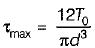Courses

# Test: Torsion of Shafts - 2

## 10 Questions MCQ Test Topicwise Question Bank for Mechanical Engineering | Test: Torsion of Shafts - 2

Description
This mock test of Test: Torsion of Shafts - 2 for Mechanical Engineering helps you for every Mechanical Engineering entrance exam. This contains 10 Multiple Choice Questions for Mechanical Engineering Test: Torsion of Shafts - 2 (mcq) to study with solutions a complete question bank. The solved questions answers in this Test: Torsion of Shafts - 2 quiz give you a good mix of easy questions and tough questions. Mechanical Engineering students definitely take this Test: Torsion of Shafts - 2 exercise for a better result in the exam. You can find other Test: Torsion of Shafts - 2 extra questions, long questions & short questions for Mechanical Engineering on EduRev as well by searching above.
QUESTION: 1

### 200 kW is to be transmitted by each of two separate shafts. A is running at 300 rpm and B at 350 rpm. Which shaft must have greater diameter?

Solution: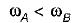As power is same,
TA > TB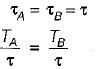or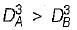∴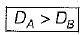QUESTION: 2

### A circular bar AB of length L is fixed at end A and free at B. Torque T is acting simultaneously at B and C. What is the strain energy U stored in the bar?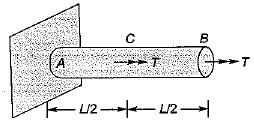Solution:

Torque on part BC = T
Torque on part AC = 2T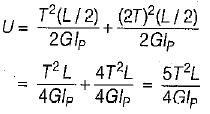QUESTION: 3

### For a power transmission shaft transmitting power P at N rpm, the diameter is proportional to

Solution: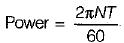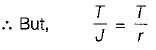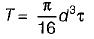∴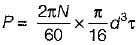∴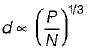QUESTION: 4

If a shaft is rotating at N revolutions per minute with an applied torque TN-m, the power being transmitted by the shaft in watt is

Solution: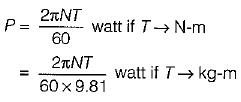QUESTION: 5

For a circular shaft of diameter d subjected to torque T, the maximum value of the shear stress is

Solution: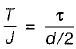⇒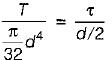∴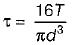QUESTION: 6

What is the maximum torque transmitted by a hollow shaft of external radius R and internal radius r?

Solution: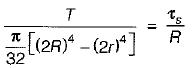∴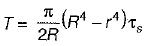QUESTION: 7

A solid shaft of circular cross-section is subjected to a torque T which produces a maximum shear stress τs in the shaft. The diameter of the shaft should be

Solution:

From the equation of torsion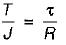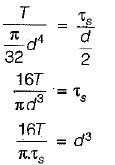⇒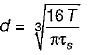QUESTION: 8

The ratio of torque carrying capacity of solid shaft to that of a hollow shaft is given by
where K is ratio of inside to outside diameter?

Solution:

τ should be same for both hollow and solid shaft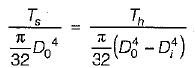⇒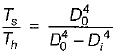⇒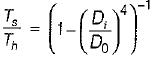∴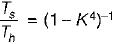QUESTION: 9

A shaft subjected to torsion experiences a pure shear stress τ on the surface. The maximum principal stress on the surface which is at 45° to the axis will have a value of

Solution: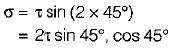QUESTION: 10

A solid shaft of diameter, d and length, L is fixed at both the ends. A torque T0 is applied at a distance L/4 from the left end as shown in figure given below.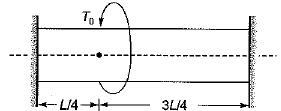The maximum shear stress in the shaft is

Solution: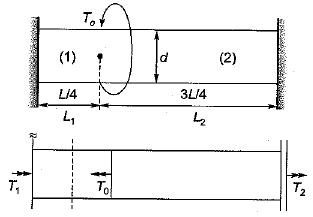T1 + T2 = T0        ......(i)
From the equation of torsion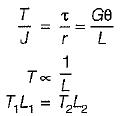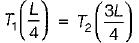T1 = 3T2         ......(ii)
From equation (i) and (ii)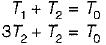⇒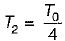and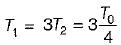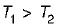Shear stress τ ∝T
Therefore maximum shear stress in the shaft witi be due to torque T1.
⇒